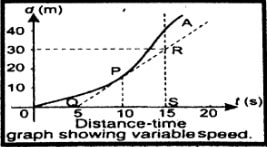What would be the shape of a speed-time graph of a body moving with variable speed?

Difficulty: Easy

An object moving with variable speed:

When an object does not cover equal distances in equal intervals of time then its speed is not constant. In this case, the distance-time graph is not a straight line.

The slope of the curve at any point can be found from the slope of the tangent at that point. For example,

the slope of the tangent at $P=\frac{RS}{QS}$

$=\frac{30m}{10s}$

$=\frac{3m}{s}$

Thus, the speed of the object at P is $\frac{3m}{s}$The speed is higher at instants when the slope is greater, speed is zero at instants when the slope is horizontal.